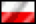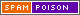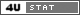# Ewa Schmeidel D.Sc., Ph.D.

## Curriculum Vitae - publications

### Scientific works:

1. LINKJ.Diblík, E.Schmeidel, On the existence of solutions of linear Volterra difference equations asymptotically equivalent to a given sequence, Applied Mathematics and Computation, 218 (2012), 9310–9320.
2. LINKE.Schmeidel, Z.Zbąszyniak, An application of Darbo's fixed point theorem in the investigation of periodicity of solutions of difference equations, Computers & Mathematics with Applications (in press).
3. LINKJ.Diblík, M.Růžičková, E.Schmeidel, M.Zbąszyniak, Weighted Asymptotically Periodic Solutions of Linear Volterra Difference Equations, Abstract and Applied Analysis (2011), 370982.
4. LINKJ.Diblik, M.Ružičková, E.Schmeidel, Asymptotically periodic solutions of Volterra systems of difference equations, Computers and Mathematics with Applications, 59 (2010), 2854-2867.
5. LINKE.Schmeidel, Properties of solutions of higher order difference equations, Vědecké Spisy Vysokého Učeni Technického v Brniě, Edice habilitační a inaugurační spisy, sv. 370, 2010.
6. LINKE.Schmeidel, Properties of solutions of higher order difference equations, Publishing House of Poznan University of Technology, 2010.
7. LINKE.Schmeidel, Boundedness of solutions of nonlinear three-dimensional difference systems with delays, Fasciculi Mathematici, 44 (2010), 107-113.
8. LINKE.Schmeidel, Oscillation of nonlinear third-dimensional difference systems with delays, Mathematica Bohemica, 135 (2010), 163-170.
9. LINKJ.Diblik, M.Ružičková, E.Schmeidel, Existence of asymptotically periodic solutions of Volterra system difference equations, Journal of Difference Equations and Applications 15 (2009), 1165–1177.
10. LINKJ.Diblik, M.Ružičková, E.Schmeidel, Asymptotically periodic solutions of Volterra difference equations, Tatra Mountains Mathematical Publications 43 (2009), 43-61.
11. E.Schmeidel, J. Elyseeva, Generalized Kiguradze's lemma on time scales with application for oscillation of higher order nonlinear dynamic equations, Fundamental'nye Fiziko-Matematiceskie Problemy i Modelirovanie Tehniko-Tehnologiceskih Sistem 11 (2008), 18-21.
12. E.Schmeidel, M. Zbąszyniak, Asymptotic behavior of solutions of third order difference equations, Proceedings of the Twelfth International Conference on Difference Equations and Applications (to appear).
13. E.Schmeidel, Asymptotic trichotomy of solutions of a class of even order nonlinear neutral difference equations with quasidifferences, Proceedings of the International Conference on Difference Equations, Special Functions and Orthogonal Polynomials, World Scientific Publishing Co (2007), 600-609.
14. LINKE.Schmeidel, J.Schmeidel, Asymptotic behavior of solutions of a class of fourth order nonlinear neutral difference equations with quasidifferences, Tatra Mountains Mathematical Publications 38 (2007), 243–254.
15. LINKE.Schmeidel, K.Janglajew, Periodic and asymptotically periodic solutions of linear difference equations, Ruffing, A. (ed.) et al., Communications of the Laufen colloquium on science, Laufen, Austria, April 1--5, 2007. Aachen: Shaker. Berichte aus der Mathematik, 14 (2007), 1-11.
16. J.Diblik, M.Ružičková, E.Schmeidel, Asymptotically periodic solutions of Volterra difference equations, Ruffing, A. (ed.) et al., Communications of the Laufen colloquium on science, Laufen, Austria, April 1--5, 2007. Aachen: Shaker. Berichte aus der Mathematik, 5 (2007), 1-12.
17. LINKE.Schmeidel, M.Migda, A.Musielak, Oscillatory of fourth order nonlinear difference equations with quasidifferences, Opuscula Mathematica 26, 2 (2006), 371-380.
18. LINKJ.Mikołajski, E.Schmeidel, Comparison of properties of solutions of differential equations and recurrence equations with the same characteristic equation, Opuscula Mathematica 26, 2 (2006), 343-349.
19. LINKJ.Diblík, M.Migda, E.Schmeidel, Bounded solutions of nonlinear discrete equations, Nonlinear Analysis 65, 4 (2006), 845-853.
20. LINKE.Schmeidel, Oscillatory and asymptotically zero solutions of third order difference equations with quasidifferences, Opuscula Mathematica 26, 2 (2006), 361-369.
21. E.Schmeidel, Asymptotic behavior of certain second order nonlinear difference equations, Proceedings of the Eight International Conference on Difference Equations and Applications, edited by S.Elaydi, G.Ladas, B.Aulbach, O.Dosly, CRC, Boca Raton, FL, (2005), 245-252.
22. M.Migda, A.Musielak, E.Schmeidel, Oscillation theorems for a class of fourth order nonlinear difference equations, Proceedings of the Eight International Conference on Difference Equations and Applications, edited by S.Elaydi, G.Ladas, B.Aulbach, O.Dosly, CRC, Boca Raton, FL, (2005), 201-208.
23. LINKE.Schmeidel, Asymptotic trichotomy for positive solutions of a class of fourth order nonlinear neutral difference equations with quasidifferences, Nonlinear Analysis 63 (2005), e899-e907.
24. M.Migda, E.Schmeidel, M.Zbaszyniak, On the existence of solutions of some second order nonlinear difference equations, Archivum Mathematicum 42 (2005), 379-388.
25. E.Schmeidel, Nonoscillation and oscillation properties of fourth order nonlinear difference equations. Proceedings of the Sixth International Conference on Difference Equations, New Progress in Difference Equations, edited by B.Aulbach, S.Elaydi, G.Ladas, CRC, Boca Raton, FL, (2004), 531-538.
26. E.Schmeidel, M.Migda, M.Zbaszyniak, Some properties of solutions of second order nonlinear difference equations, Functional Differential Equations 11 (1-2) (2004), 147-152.
27. LINKM.Migda, A.Musielak, E.Schmeidel, On a class of fourth order nonlinear difference equations, Advances in Difference Equations 1 (2004), 23-36.
28. J.Jackowski, E.Schmeidel, G.Schmeidel, Komputerowy program prognozowania porowatosci w metalowych odlewach kompozytowych z nasycanym zbrojeniem, Archiwum Odlewnictwa 4(11) (2004), 200-208.
29. E.Schmeidel, Nonoscillation properties of certain fourth order nonlinear difference equations, Functional Differential Equations 11 (1-2) (2004), 141-146.
30. M.Migda, E.Schmeidel, Asymptotic properties of fourth order nonlinear difference equations, Mathematical and Computer Modelling 39 (2004), 1203-1211.
31. E.Schmeidel, Nonoscillation and oscillation theorems for a fourth order nonlinear difference equations, Fields Institute Communications 42 (2004), 313-321.
32. E.Schmeidel, Oscillation and nonoscillation theorems for fourth order difference equations, Rocky Mountain Journal of Mathematics, 33 (3) (2003), 1083-1094.
33. M.Migda, E.Schmeidel, A.Drozdowicz, Nonoscillation results for some third order nonlinear difference equation, Folia Facultatis Scientiarium Naturalium Universitatis Masarykianae Brunensis Mathematica 13 (2003), 185-192.
34. E.Schmeidel, On the asymptotic behavior of solutions of second order nonlinear difference equations, Folia Facultatis Scientiarium Naturalium Universitatis Masarykianae Brunensis Mathematica 13 (2003), 287-293.
35. J.Jackowski, E.Schmeidel, Model kształtowania się porowatosci w nasycanych odlewach kompozytowych, Archiwum Technologii Maszyn i Automatyzacji 23(1) (2003), 71-83.
36. E.Schmeidel, M.Migda, E.Magnucka-Blandzi, Mathematical works of Jerzy Popenda, Applied Mathematics E-Notes 2 (2002), 155-170.
37. M.Migda, E.Schmeidel, On the asymptotic behaviour of solutions of nonlinear difference equations, Fasciculi Mathematici 31 (2001), 63-69.
38. LINKE.Schmeidel, B.Szmanda, Oscillatory and asymptotic behavior of certain difference equations, Nonlinear Analysis, Theory, Methods & Applications 47 (2001), 4731-4742.
39. E.Schmeidel, Jerzy Popenda (1948-1999), Wiadomosci Matematyczne 36 (2000), 185-189.
40. M.Migda, E.Schmeidel, Some properties of solutions of a class of nonlinear difference equations, Proceedings of the International Conference on Differential Equations-Berlin 1999, World Scientific, Singapore(2000), 1476-1478.
41. J.Popenda, E.Schmeidel, On the asymptotically periodic solution of some linear difference equations, Archivum Mathematicum 35, 1 (1999), 13-19.
42. M.Migda, E.Schmeidel, Some properties of solutions of a class of nonlinear difference equations, Acta Univ. Palacki, Mathematica 38 (1999), 131-137.
43. J.Popenda, E.Schmeidel, Asymptotically periodic solution of some linear difference equations, Facta Universitatis, Mathematics and Informatics , 14 (1999), 31-40.
44. M.Migda, E.Schmeidel, Asymptotic behavior of solutions of higher order difference equations, Demonstratio Mathematica 31, 1 (1998), 71-80.
45. M.Migda, E.Schmeidel, Some properties of solutions of a class of nonlinear difference equations, ICDEA'98, (1998), 257-260.
46. E.Schmeidel, On the asymptotic behaviour of solutions of difference equations, Demonstratio Mathematica 30, 1 (1997), 193-197.
47. E.Schmeidel, Asymptotic properties of solution of nonlinear difference equation, Communications of Applied Nonlinear Analysis 2, 4 (1997), 87-92.
48. LINKJ.Popenda, E.Schmeidel, On the asymptotic behaviour of solutions of integer valued difference equations, Nonlinear Analysis, Theory, Methods & Applications 30, 2 (1997), 1119-1124.
49. J.Popenda, E.Schmeidel, On the asymptotic behavior of solutions of nonhomogeneous linear difference equations, Indian Journal of Pure and Applied Mathematics 28, 3 (1997), 319-327.
50. LINKJ.Popenda, E.Schmeidel, On the solutions of fourth order difference equations, Rocky Mountain Journal of Mathematics 25, 4 (1995), 1485-1499.
51. LINKJ.Popenda, E.Schmeidel, On the asymptotic behavior of solutions of linear difference equations, Publicacions Matematiques 38 (1994), 3-9.
52. E.Schmeidel, Asymptotic behaviour of solutions of the second order difference equation, Demonstratio Mathematica 26, 3-4 (1993), 811-819.
53. J.Popenda, E.Schmeidel, Nonoscillatory solutions of third order difference equations, Portugaliae Mathematica 49, 2 (1992), 233-239.
54. J.Popenda, E.Schmeidel, Some properties of solutions of difference equations, Fasciculi Mathematici 13 (1981), 89-98.
55. J.Popenda, E.Schmeidel, On the asymptotic behaviour of nonoscillatory solutions of difference equations, Fasciculi Mathematici 12 (1980), 43-53.

news

Last update : 2012-08-15 UTC 07:53:56
Contact | ©2011 Szymon & Opensite | Project and realization: Szymon & Yunnan ||